When importing multiple CSV files that reference each other through various IDs, you may want more granular control.

Instead of looking at and modifying the YAML file in a text editor: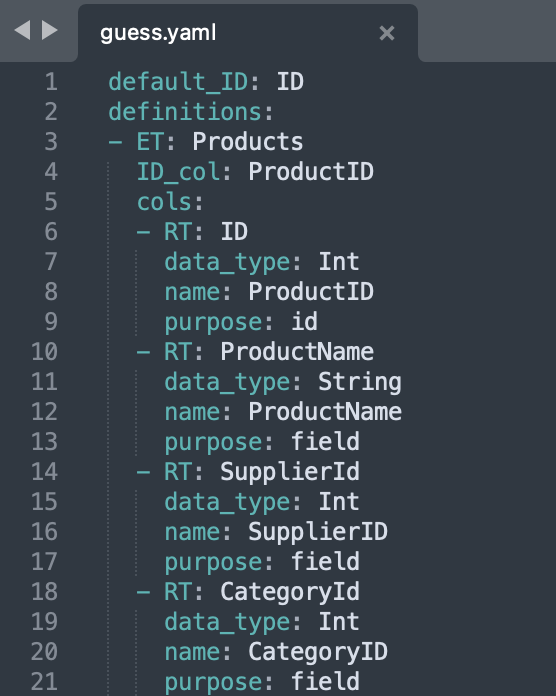You can use our graphical ImGui interface!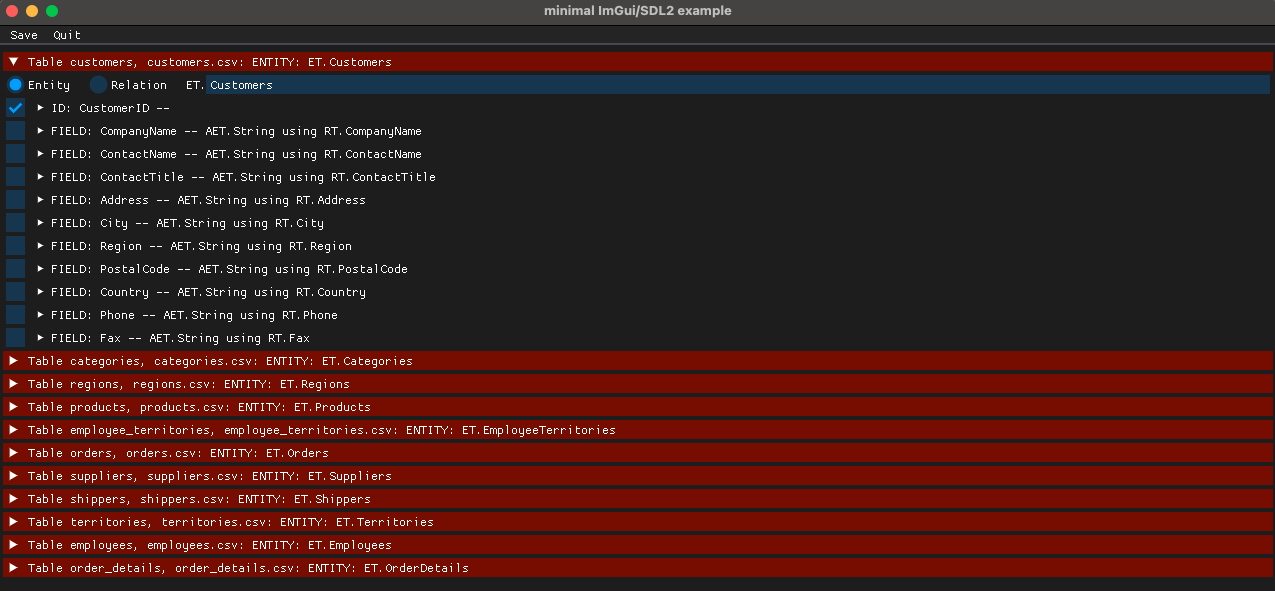If you're using MacOS, make sure you use Python 3.9 (as of March 2022, Python 3.10 does not play nicely with ImGui).

In this example, we'll use the Northwind dataset. We've made the zipped CSV files available northwind.zip.

Also check out our blog post on SQL imports.

## Install ImGui and SDL2​

pip3 install "pyimgui[sdl2]"brew install SDL2

## Generate YAML file​

from zef import *from zef.ops import *from zef.experimental import sql_importdecl = sql_import.guess_csvs("*.csv")decl | write_file["sql_import.yaml"] | run

## Open YAML file with ImGui interface​

If using MacOS, make sure you use Python 3.9 (as of March 2022) and are using the Python 3.9 Zef wheel.

python3.9 -m zef.experimental.sql_ui.wizard sql_import.yaml

## Change Entity names from plural to singular​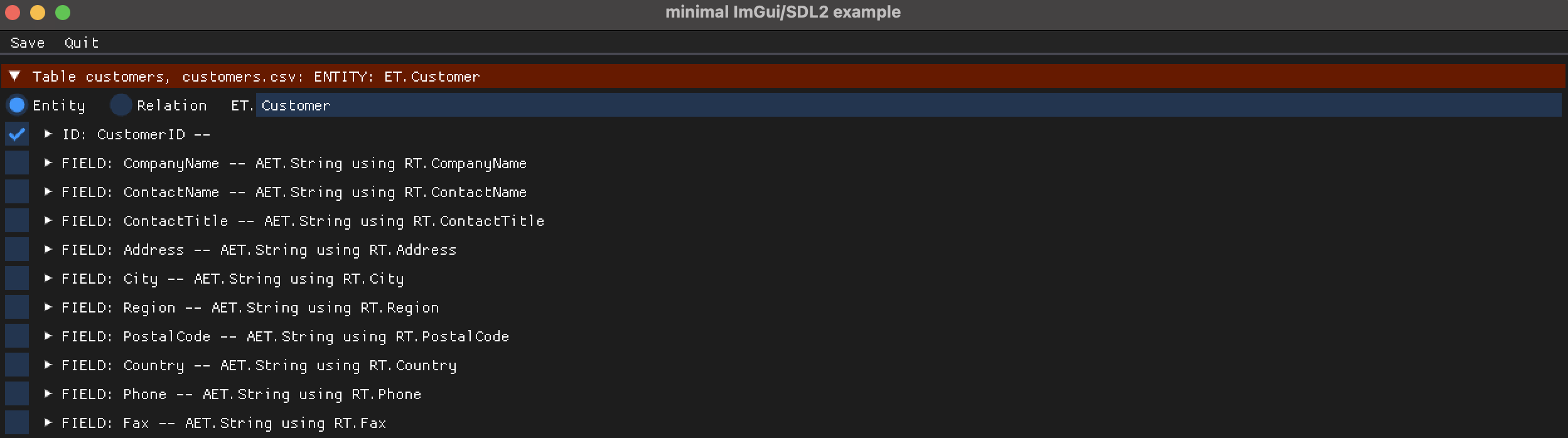## Change cross-referenced IDs from Field to Entity and rename their RTs​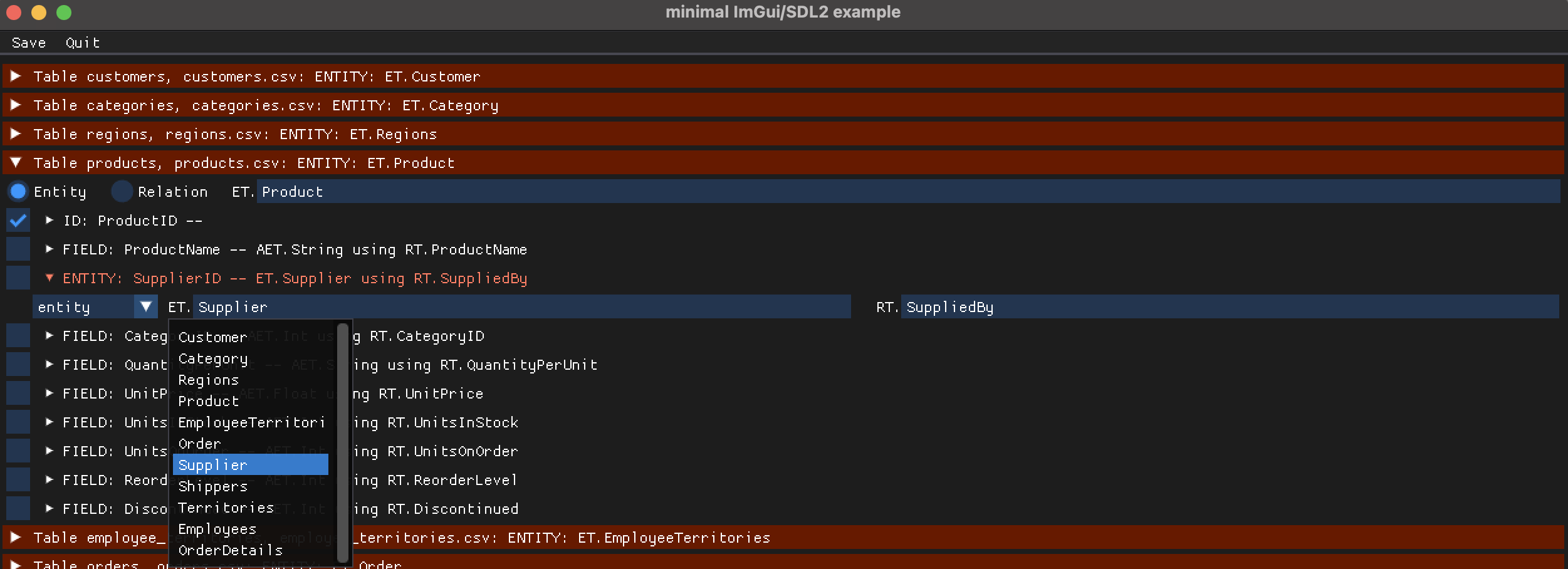See your options through the dropdown (make sure the Entity names are edited first to see the correct options).

## Change AET types​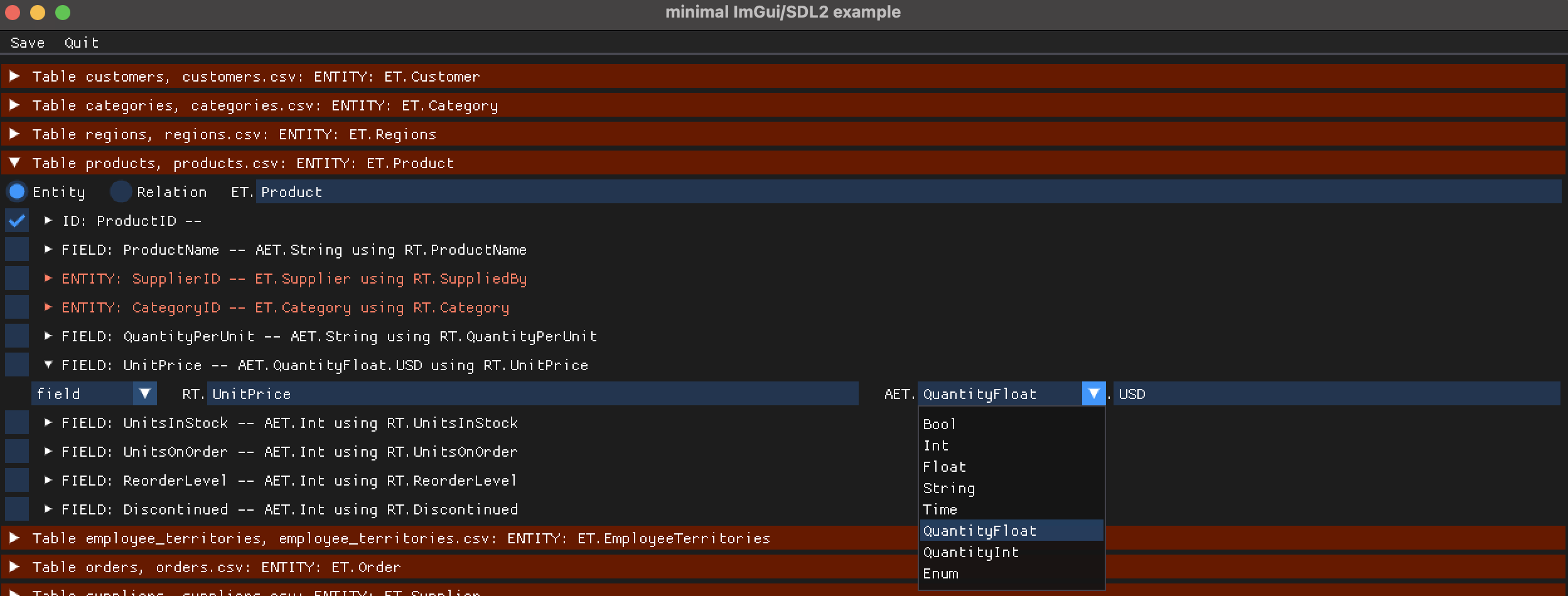## Change Entities to Relations​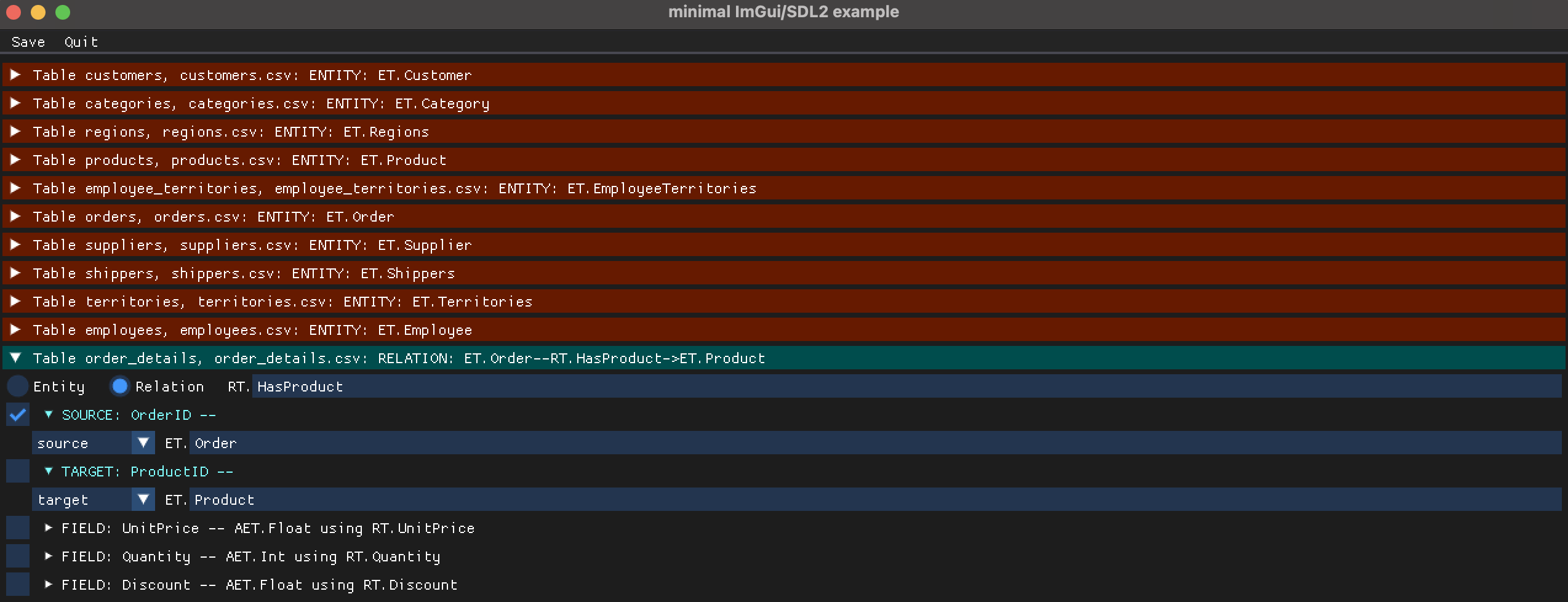Set the correct Source and Target.

## Save your edited YAML file​

The edited YAML results will save to the current filename.

If needed, we may add an option to save to a new filename in the future.

## Load edited YAML file into Zef​

decl = "sql_import.yaml" | load_file | run | get["content"] | collectg = Graph()actions = sql_import.import_actions(decl)actions | transact[g] | run

See the results of your import!

yo(g)<...snip...>^^^^^^^^^^^^^^^^^^^^^^^^^^^^^^^^^^^^^^^^^ Atomic Entities ^^^^^^^^^^^^^^^^^^^^^^^^^^^^^^^^^^^^^^^[6007 total, 6007 alive]       AET.String[2985 total, 2985 alive]       AET.Float[2527 total, 2527 alive]       AET.Int[2232 total, 2232 alive]       AET.QuantityFloat.dollars[2469 total, 2469 alive]       AET.Time^^^^^^^^^^^^^^^^^^^^^^^^^^^^^^^^^^^^^^^^^^^^ Entities ^^^^^^^^^^^^^^^^^^^^^^^^^^^^^^^^^^^^^^^^^^^[1660 total, 1660 alive]       ET.Order[154 total, 154 alive]         ET.Product[12 total, 12 alive]           ET.Employee[93 total, 93 alive]           ET.Customer[53 total, 53 alive]           ET.Territory[4 total, 4 alive]             ET.Region[3 total, 3 alive]             ET.Shipper[29 total, 29 alive]           ET.Supplier[8 total, 8 alive]             ET.Category^^^^^^^^^^^^^^^^^^^^^^^^^^^^^^^^^^^^^^^^^^^  Relations ^^^^^^^^^^^^^^^^^^^^^^^^^^^^^^^^^^^^^^^^^^[4 total, 4 alive]             RT.RegionDescription       [4 total, 4 alive]             (ET.Region, RT.RegionDescription, AET.String)[2155 total, 2155 alive]       RT.Order       [2155 total, 2155 alive]       (ET.Order, RT.Order, ET.Product)[9 total, 9 alive]             RT.ReportsTo       [9 total, 9 alive]             (ET.Employee, RT.ReportsTo, ET.Employee)<...snip...>